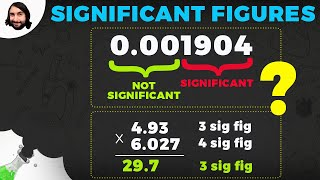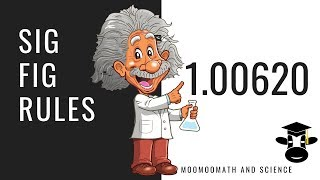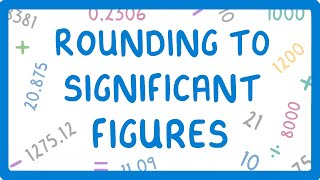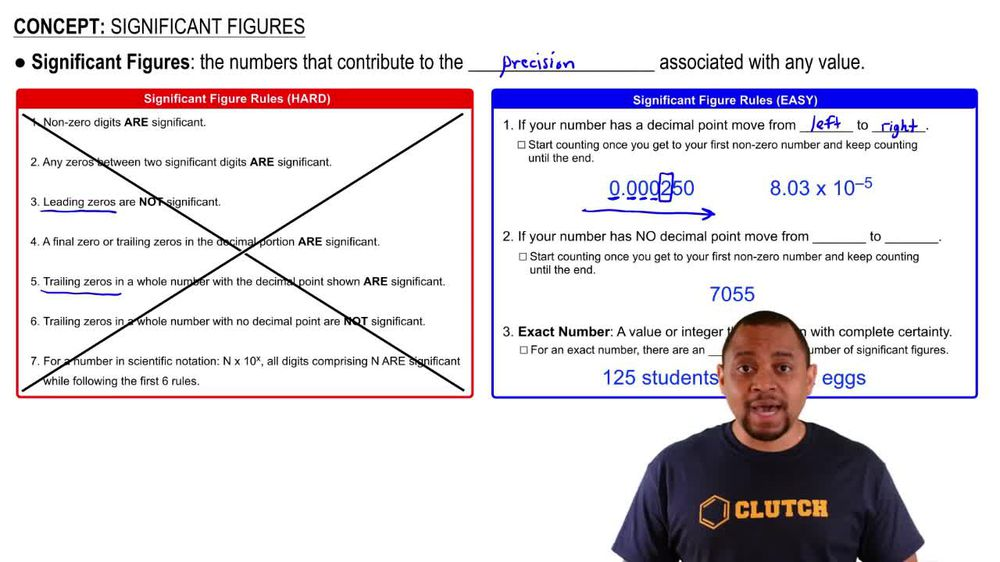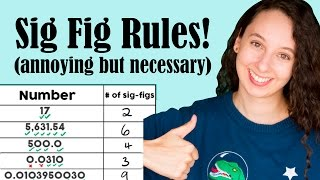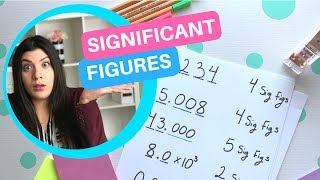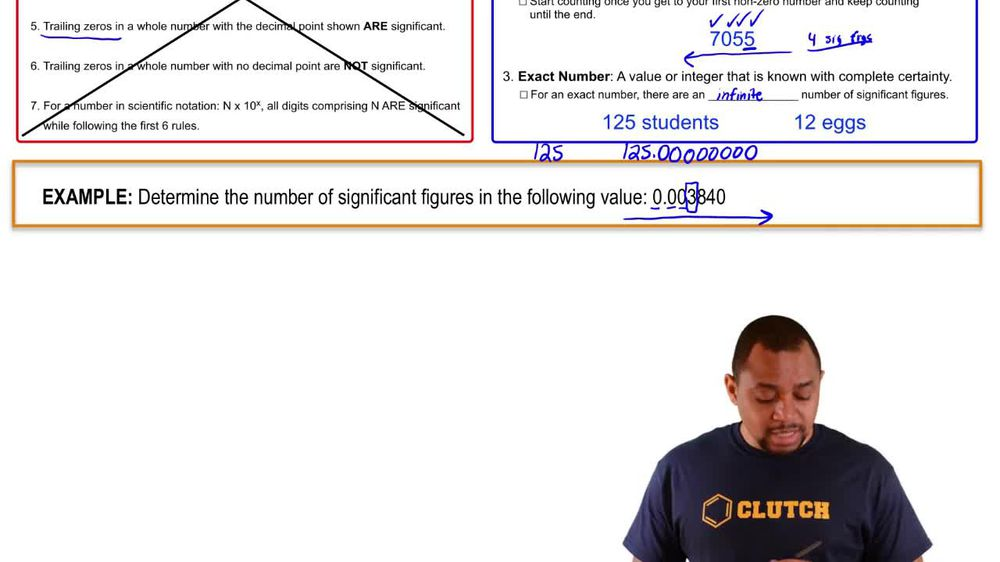Start typing, then use the up and down arrows to select an option from the list.
1. 1. Intro to General Chemistry2. Significant Figures
Problem

# What is the number of significant figures in each of the following measured quantities? (e) 92.03 km

Relevant Solution1m
Play a video:
Hi everyone for this problem, we need to determine the number of significant figures in 6.92300 times 10 to the third yard. So this is written a little weird. So let's go ahead and write this out all the way. So when we have 10 to the third, that means we're moving the decimal place three units to the right. So we have 123. And so when we write this out, it's really yards. Okay. And so for this we have all non zero digits and all non zero digits in a measurement are significant. And so since we only have four, our final answer here is going to be four significant figures. And that is the answer to this problem. There are only four significant figures and were able to figure that out by writing out our Our number all the way and taking out the power. And when we did that we got 6,923 yards. That's the end of this problem. I hope this was helpful.